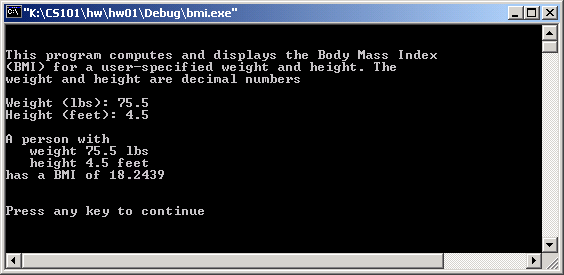# CS 101 Homework 2 Assigned in Laboratory 2 Due at start of Laboratory 3

## Pledge

• This assignment is pledged. You may discuss its content and your approach with anyone, but you must do all your own work. You must create the logic of the solution, type your own code, and compile, execute, and debug your own program. You may not use code developed by anyone else.

## Objective

• The object of this assignment is two-fold:
• To practice coding programs that do interactive input and output. To illustrate how you write a program that computes useful data.

## Problem

• Good programmers often write short programs that compute useful things. For example, a value of interest to many people is their body mass index (BMI). BMI is a function of a person’s weight and height and is used as a healthiness measure. Your program will compute and display the BMI for a user-specified weight and height. Because BMI is normally expressed metrically, the person’s weight and height are first converted from the English system of pounds and feet to kilograms and meters respectively. Computing the BMI is then a relatively straightforward calculation—it is the ratio of weight to the square of the height.
• Your program's name must should be bmi.cpp
• All numeric values should be double-precision floating points.

## Program

• We suggest the following steps in the program
• Define constants
• Display program legend
• Prompt user for the weight of interest
• Extract the weight
• Prompt user for the height of interest
• Extract the height
• Compute metric equivalents of input values
• Note there are 0.454 kilograms per pound and  0.3046 meters per foot
• Perform BMI calculation
• Display result

## Submissions

• From now on, use the following as the standard header of every homework you submit must begin with the following material:
`// Programmer: `
`// Student ID:`
`// E-mail: `
`// CS101 lab section number:`
`// CS101 lab section time:`
`// Pledge: On my honor as a student of the University of Virginia, I`
`// have neither given nor received unauthorized help (as defined in`
`// this assignment) on this homework. <type your name here>`
• Print out your source code, sign the pledge with your handwritten signature near where you typed your name, staple the grading criteria sheet (available from the website) to your printout, and submit the hardcopy to your TA at the beginning of your next lab session.
• Late homework assignments are not accepted.

## Notes

• A sample of the desired execution is shown below. Observe the title, warning legend, the nice prompts, and the labeled output. While you do not have to use exactly the phrasing seen below, your output must be of equal or higher quality.• For your input and output, you must use the standard iostream library. Do not use any console or graphical libraries.
• You must use our formulas and process above for this problem. We know there are non-metric formulas for this problem, but do not use them. The purpose of this homework is to help you understand expressions and their evaluation.
• Once more, remember that all calculations are double-precision floating-point.